Courses

# RD Sharma Solutions -Ex-20.2, Mensuration, Class 6, Maths Class 6 Notes | EduRev

## RD Sharma Solutions for Class 6 Mathematics

Created by: Abhishek Kapoor

## Class 6 : RD Sharma Solutions -Ex-20.2, Mensuration, Class 6, Maths Class 6 Notes | EduRev

The document RD Sharma Solutions -Ex-20.2, Mensuration, Class 6, Maths Class 6 Notes | EduRev is a part of the Class 6 Course RD Sharma Solutions for Class 6 Mathematics.
All you need of Class 6 at this link: Class 6

Question 1. Find the perimeter of the rectangle whose lengths and breadths are given below:

(i) 7 cm, 5 cm

(ii) 5 cm, 4 cm

(iii) 7.5 cm, 4.5 cm

Solution: (i) Perimeter of a rectangle = 2 × (Length + Breadth)

Since, Length = 7 cm, Breadth = 5 cm

Therefore, Perimeter = 2 × (7 + 5) = 2 × (12) = 24 cm

(ii) Perimeter of a rectangle = 2 × (Length + Breadth)

Since, Length = 5 cm, Breadth = 4 cm

Therefore, Perimeter = 2 × (5 + 4) = 2 × (9) = 18 cm

(iii) Perimeter of a rectangle = 2 × (Length + Breadth)

Since, Length = 7.5 cm, Breadth = 4.5 cm

Therefore, Perimeter = 2 × (7.5 + 4.5) = 2 × (12) = 24 cm

Question 2. Find the perimeter of the squares whose sides are given below:

(i) 10 cm (ii) 5 m (iii) 115.5 cm

Solution: Perimeter of a square = 4 x (Length of one side)

(i) Length of one side = 10 cm

Perimeter = 4 × 10 = 40 cm

(ii) Length of one side = 5 m

Perimeter = 4 × 5 = 20 m

(iii) Length of one side = 115.5 cm

Perimeter = 4 × 115.5 = 462 cm

Question 3. Find the side of the square whose perimeter is:

(i) 16 m (ii) 40 cm (iii) 22 cm

Solution: Side of a square = Perimeter 4

(i) Perimeter = 16 m

Side of this square =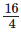= 4 m

(ii) Perimeter = 40 cm

Side of this square =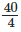= 10 cm

(iii) Perimeter = 22 cm

Side of this square =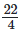= 5. 5 cm

Question 4. Find the breadth of the rectangle whose perimeter is 360 cm and whose length is

(i) 116 cm (ii) 140 cm (iii) 102 cm

Solution: Perimeter of a rectangle = 2 (Length + Breadth)

Therefore, Breadth of the rectangle =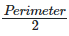- Length

(i) Perimeter = 360 cm

Length = 116 cm

Breadth =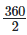- 116

= 180 - 116 = 64 cm

(ii) Perimeter = 360 cm

Length = 140 cm

Breadth =- 140

= 180 -140 = 40 cm

(iii) Perimeter = 360 cm

Length = 102 cm

Breadth =- 102 = 180 - 102 = 78 cm

Question 5. A rectangular piece of lawn is 55 m wide and 98 m long. Find the length of the fence around it.

Solution: Length of the lawn = 98 m

Breadth of the lawn = 55 m

Length of the fence around the lawn = Perimeter of the lawn = 2 × (Length + Breadth)

Perimeter of the lawn = 2 × (98 + 55) m = 2 × (153) = 306 m

Thus, the length of the fence around the lawn = 306 m

Question 6. The side of a square field is 65m. What is the length of the fence required all around it?

Solution: Side of the square field = 65 m

Length of the fence around the square field = Perimeter of the square field = 4 × (Side of the square)

Perimeter of the square field = 4 × 65 = 260 m

Thus, the length of the fence around the square filed = 260 m

Question 7. Two sides of a triangle are 15 cm and 20 cm. The perimeter of the triangles is 50 cm. What is the third side?

Solution: Given: Perimeter = 50 cm

Length of the first side = 15 cm

Length of the second side = 20 cm

We have to find the length of the third side.

Perimeter of a triangle = Sum of all three sides of the triangle

Length of the third side = (Perimeter of the triangle) - (Sum of the length of the other two sides)

= 50 - (15 + 20)

= 50 - 35 = 15 cm

Question 8. A wire of length 20 m is to be folded in the form of a rectangle. How many rectangles can be formed by folding the wire if the sides are positive integers in metres?

Solution: It is given that a wire of length 20 m is to be folded in the form of a rectangle;

Therefore, we have: Perimeter of the rectangle = 20 m

=> 2 (Length + Breadth) = 20 m

=> (Length + Breadth) = 20/2 = 10 m

Since, length and breadth are positive integers in metres, therefore, the possible dimensions are: (1m, 9m), (2m, 8m), (3m, 7m), (4m, 6m) and (5m, 5m)

Thus, five rectangles can be formed with the given wire.

Question 9. A square piece of land has each side equal to 100 m. If 3 layers of metal wire have to be used to fence it, what is the length of the wire?

Solution: Side of the square field = 100 m

Wire required to fence the square field = Perimeter of the square field = 4 × Side of the square field Perimeter = 4 × 100 = 400 m

This perimeter is the length of wire required to fence one layer.

Therefore, the length of wire required to fence three layers = 3 × 400 m = 1200 m

Question 10. Shikha runs around a square of side 75m. Priya runs around a rectangle with length 60 m and breadth 45 m. Who covers the smaller distance?

Solution: Shikha and Priya, while running around the square and rectangular field respectively, actually cover a distance equal to the perimeters of these fields.

Distance covered by Shikha = Perimeter of the square = 4 × 75 m = 300 m

Similarly, distance covered by Priya = Perimeter of the rectangle = 2 × (60 + 45)

= 2 × 105 = 210 m

Thus, it is evident that the distance covered by Priya is less than that covered by Shikha.

Question 11. The dimensions of a photographs are 30 cm X 20 cm. What length of wooden frame is needed to frame the picture?

Solution: Dimensions of the photograph = 30 cm x 20 cm

So, the required length of wooden frame = Perimeter of the photograph

= 2 × (30 + 20) cm = 2 × 50 cm

= 100 cm

Question 12. The length of a rectangular field is 100 m. If its perimeter is 300 m, what is its breadth?

Solution: Length of the rectangular field = 100 m

Perimeter of the rectangular field = 300 m

Perimeter of a rectangle = 2 (Length + Breadth)

Applying the above formula, we get:

Breadth of the rectangular field =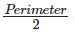- Length

=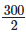- 100

= 150 - 100 = 50 m

Question 13. TO fix fence wires in a garden. 70 m long and 50 m wide, Arvind bought metal pipes for posts. He fixed a post every 5 metres apart; each post was 2 m long. What is total length og the pipers he bought for the posts?

Solution: Length of the garden = 70 m

Breadth of the garden = 50 m

Perimeter of the garden = 2 × (Length + Breadth)

= 2 × (70 + 50)

= 2 × 120 = 240 m

On the perimeter of the garden, it is given that Arvind fixes a post every 5 metres apart.

So, the number of posts required =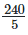= 48

Since, Length of each post = 2 m

Therefore, Total length of the pipe required = 48 × 2 = 96 m

Question 14. Find the cost of fencing a rectangular park of length 175 m and breadth 125 m at the rate of Rs 12 per meter.

Solution: Length of the park = 175 m

Breadth of the park = 125 m

Perimeter of the park = 2 × (Length + Breadth)

= 2 × (175 + 125)

= 2 × 300 = 600 m

Rate of fencing = Rs. 12 per meter

Cost of fencing = Rs. 12 × 600 = Rs. 7,200

Question 15. The perimeter of a rectangular pentagon is 100 cm. How long is each side?

Solution: A regular pentagon is a closed polygon having five sides of equal length.

Perimeter of the regular pentagon = 100 cm

Perimeter of the regular pentagon = 5 x Side of the regular pentagon

Therefore, side of the regular pentagon = Perimeter / 5

= 100 / 5 = 20 cm

Question 16. Find the perimeter of a regular hexagon with each side measuring 8 m.

Solution: A regular hexagon is a closed polygon having six sides of equal lengths.

Side of the hexagon = 8 m

Perimeter of the hexagon = 6 × Side of the hexagon

= 6 × 8 = 48 m

Question 17. A rectangular piece of land measure 0.7 km by 0.5 km. Each side is to be fenced with four rows of wires. What length of the wire is needed?

Solution: Dimensions of the rectangular land = 0.7 km x 0.5 km

Perimeter of the rectangular land = 2 (Length + Breadth)

= 2 (0.7 + 0.5) km

= 2 × 1.2 km = 2.4 km

This perimeter is equal to one row of wire required to fence the land.

Therefore, length of wire required to fence the land with four rows of wire = 4 × 2.4 km

= 9.6 km

Question 18. Avneet buys 9 square paving slabs, each with a side of ½ m. He lays them in the form of a square.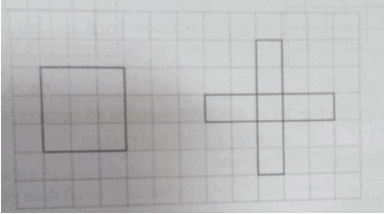(i) What is the perimeter of his arrangement?

(ii) Shari does not like his arrangement. She gets him to lay them out like a cross. What is the perimeter of her arrangement?

(iii) Avneet wonders, if there is a way of getting an even greater perimeter. Can you find a way of doing this? (The paving slabs must meet along complete edges they cannot be broken)

Solution: (i) Length of the side of one slab = 1/2 m

In the square arrangement, one side of the square is formed by three slabs.

So, length of the side of the square = 3 × ½ = 3/2 m

The perimeter of the square arrangement = 4 × 3/2 = 6 m

(ii) The cross arrangement consists of 8 sides.

These sides form the periphery of the arrangement and measure 1 m each.

Also, this arrangement consists of other 4 sides that measure 1/2 m each.

So, the perimeter of the cross arrangement = (1 + 1/2 + 1 + 1 + 1/2 + 1 + 1 + 1/2 + 1 + 1 + 1/2 + 1)

= (8 + 2) = 10 m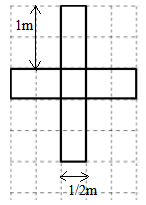(iii) Perimeter of the cross arrangement = 10 m

Perimeter of the square arrangement = 6 m

Thus, the perimeter of the cross arrangement is more than that of the square arrangement.

(iv) No, there is no way of arranging these slabs where the perimeter is more than 10 m.

103 docs

,

,

,

,

,

,

,

,

,

,

,

,

,

,

,

,

,

,

,

,

,

,

,

,

,

,

,

,

,

,

;